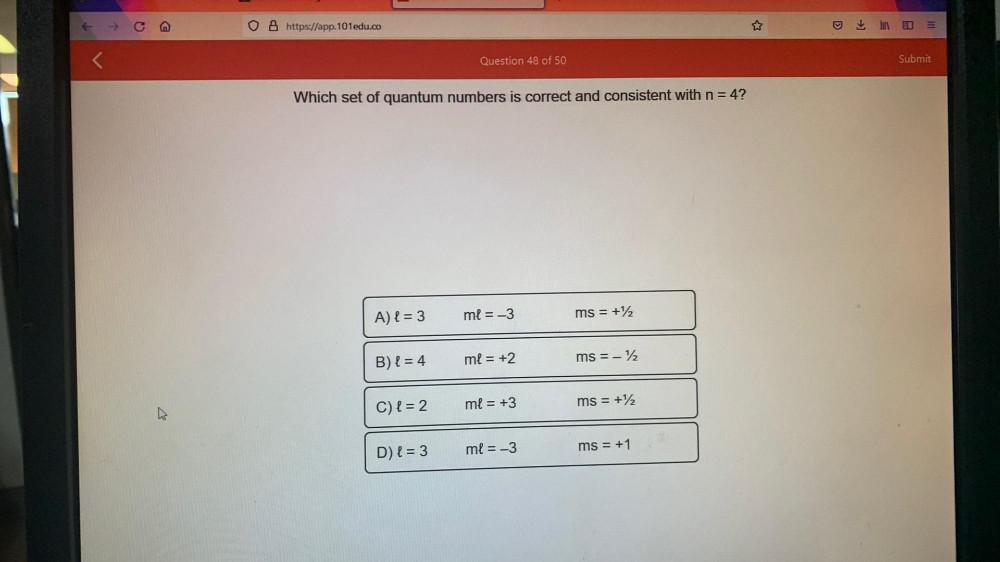Question:

# Which set of quantum numbers is correct and consistent with

Last updated: 7/28/2022Which set of quantum numbers is correct and consistent with n = 4? A) l = 3 ml = -3 ms = +½ B) l = 4 ml = +2 ms=-½ C) l = 2 ml = +3 ms =+½ D) l = 3 ml = -3 ms = +1